Courses

# Solutions of Electricity (Page No- 39) - Physics By Lakhmir Singh, Class 10 Class 10 Notes | EduRev

## Class 10 : Solutions of Electricity (Page No- 39) - Physics By Lakhmir Singh, Class 10 Class 10 Notes | EduRev

The document Solutions of Electricity (Page No- 39) - Physics By Lakhmir Singh, Class 10 Class 10 Notes | EduRev is a part of the Class 10 Course Class 10 Physics Solutions By Lakhmir Singh & Manjit Kaur.
All you need of Class 10 at this link: Class 10

Lakhmir Singh Physics Class 10 Solutions Page No:39

Question 18:
Two resistors, with resistances 5 Ω and 10 Ω respectively are to be connected to a battery of emf 6 V so as to obtain :
(i) minimum current flowing (ii) maximum current flowing
(a) How will you connect the resistances in each case ? .
(b) Calculate the strength of the total current in the circuit in the two cases.

Solution :
Given: Two resistors with resistances R1=5ohm and R2=10ohm, V=6volt
(a) For minimum current these two should be connected in series. For maximum current these two should be connected in parallel.
(b)In series,
Total resistance = 5+10 = 15ohms
Therefore total current drawn = V/R = 6/15 = 0.4amps
In parallel,
Total resistance R is given as
1/R=1/R1+1/R2
1/R=1/5+1/10
1/R=3/10
R=10/3ohm
Therefore total current drawn by the circuit = V/R = 6/(10/3) =1.8amps.

Question 19:
The circuit diagram given below shows the combination of three resistors R1 R2 and R3 :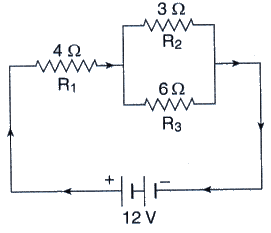Find : (i) total resistance of the circuit.
(ii) total current flowing in the circuit.
(iii) the potential difference across R1.

Solution :
(i) Total resistance of two resistors that are connected in parallel is
1/R’ = 1/3+1/6
1/R’ = 3/6
R’ = 2ohms
Total resistance of the circuit = 2+4ohms = 6ohms
(ii) Total current flowing through the circuit=V/total resistance
I = 12/6 = 2amps
(iii) Potential difference across R1=R1 x I = 4 x 2 = 8V.

Question 20:
In the circuit diagram given below, the current flowing across 5 ohm resistor is 1 amp. Find the current flowing through the other two resistors.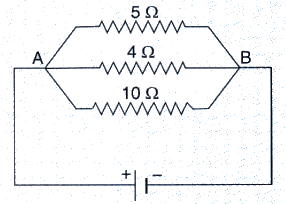Solution :
Given :-
1 amp current is flowing through 5ohm resistor.
We know that in case of parallel connection, the p.d. across each resistor is same and is equal to the voltage applied.
Therefore, applied voltage, V = IR = 1 x 5 = 5V
So,
Current through 4 ohm resistor = V/R = 5/4 = 1.25 A
Current through 10 ohm resistor = V/R = 5/10 = 0.5 A

Question 21:
A resistor has a resistance of 176 ohms. How many of these resistors should be connected in parallel so that their combination draws a current of 5 amperes from a 220 volt supply line ?
Solution :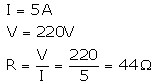Required resistance is less than 176Ω, so the resistors should be connected in parallel. Let the required no. be n.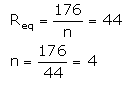Question 22:
An electric heater which is connected to a 220 V supply line has two resistance coils A and B of 24 Ω resistance each. These coils can be used separately (one at a time), in series or in parallel. Calculate the current drawn when :
(a) only one coil A is used.
(b) coils A and B are used in series.
(c) coils A and B are used in parallel.

Solution :

Given V = 220V
RA = RB = 24 ohm
(a) Current drawn when only coils A and B used :
I = V/RA = 220/24

= 9.16amps

(b) Current drawn when coils A and B are used in series:
Total resistance. R = RA + RB = 24+24 = 48ohms
I = V/R = 220/48 = 4.58amps

(c) Current drawn when coils A and B are used in parallel:

Total resistance. 1/R = 1/R+ 1/RB = 1/24 + 1/24 = 2/24 = 1/12

R = 12ohms

I = V/R = 220/12

= 18.33amps

Question 23:
In the circuit diagram given below five resistances of 10 Ω, 40 Ω, 30 Ω, 20 Ω and 60 Ω are connected as shown to a 12 V battery.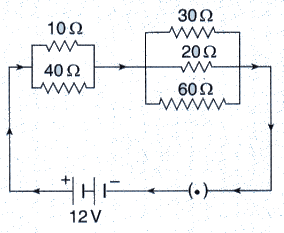Calculate :
(a) total resistance in the circuit.
(b) total current flowing in the circuit.

Solution :

(i) Equivalent resistance of 10 Ω and 40 Ω reistances (connected in parallel) is R1, given as: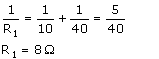Equivalent resistance of 30Ω, 20 Ω and 60 Ω reistances (connected in parallel) is R2, given as :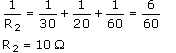R1 and R2 are connected in series.
∴ Total resistance in the circuit is R = R1 + R2 = 8 + 10 = 18 Q

(ii) Total current flowing in the circuit,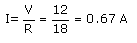Offer running on EduRev: Apply code STAYHOME200 to get INR 200 off on our premium plan EduRev Infinity!

94 docs

,

,

,

,

,

,

,

,

,

,

,

,

,

,

,

,

,

,

,

,

,

,

,

,

;